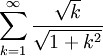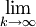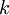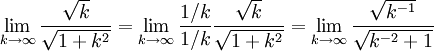# Divergence Test With Square Roots

Previous: Simple Divergence Test Example

Next: Divergence Test with arctan

## Question

Use the divergence test to determine whether the infinite seriesconverges or diverges, or if the test yields no conclusion.

## Solution

As always, we apply the divergence theorem by evaluating a limit as k tends to infinity. In this case we findTherefore, because the above limit equals zero, the divergence test yields no conclusion.

## Discussion of Each Step

### Step (1)

Essentially, we replaced the sigmain the given series with a limitto obtain the left-hand-side of (1). To obtain the right-hand-side we used a common trick for evaluating limits: diving numerator and denominator by the highest power of.### Step (2)

In the second step, we took the limits inside the radical signs:## Potential Challenge Areas

### Getting Started

Since the question asks for the divergence test to be used, getting started would imply thatneeds to be evaluated.

### Coming to the Wrong Conclusion

When the limit yields zero, the divergence test yields no conclusion. Other tests might be used to determine whether this limit diverges or converges. In this particular example, this might be difficult using only the tests we cover in the ISM. In the last lesson of this unit, we will address what one could do if all our tests fail (link to be added here).

Previous: Simple Divergence Test Example

Next: Divergence Test with arctan